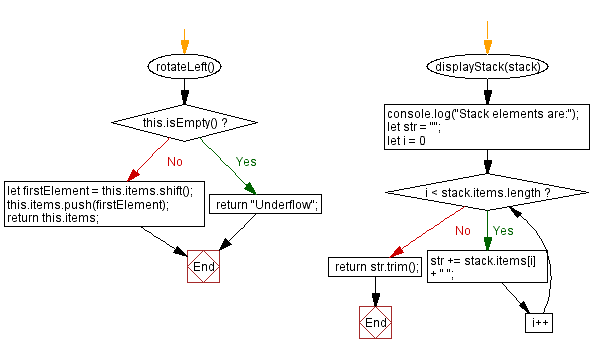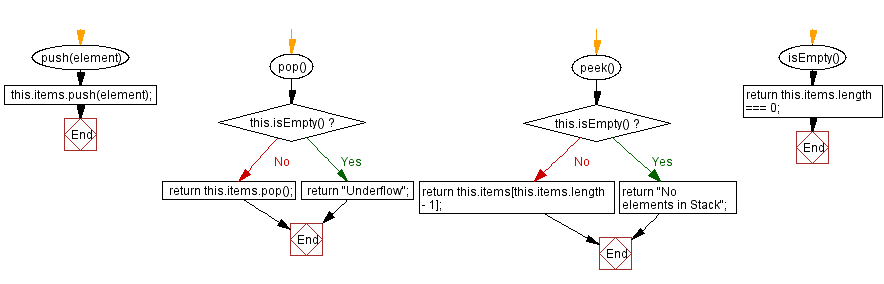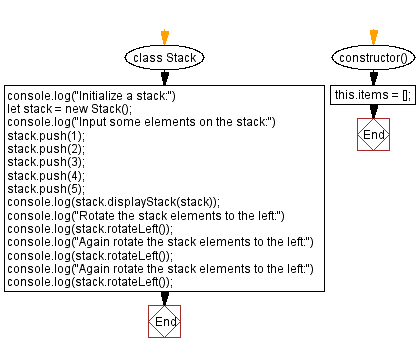# JavaScript Exercises: Rotate the stack elements to the right

## JavaScript Stack: Exercise-12 with Solution

Write a JavaScript program to rotate the stack elements to the right direction.

Sample Solution:

JavaScript Code:

``````class Stack {
constructor() {
this.items = [];
}

push(element) {
this.items.push(element);
}

pop() {
if (this.isEmpty())
return "Underflow";
return this.items.pop();
}

peek() {
if (this.isEmpty())
return "No elements in Stack";
return this.items[this.items.length - 1];
}

isEmpty() {
return this.items.length === 0;
}

rotateLeft() {
if (this.isEmpty()) {
return "Underflow";
}
let firstElement = this.items.shift();
this.items.push(firstElement);
return this.items;
}
displayStack(stack) {
console.log("Stack elements are:");
let str = "";
for (let i = 0; i < stack.items.length; i++)
str += stack.items[i] + " ";
return str.trim();
}
}
console.log("Initialize a stack:")
let stack = new Stack();
console.log("Input some elements on the stack:")
stack.push(1);
stack.push(2);
stack.push(3);
stack.push(4);
stack.push(5);
console.log(stack.displayStack(stack));
console.log("Rotate the stack elements to the left:")
console.log(stack.rotateLeft());
console.log("Again rotate the stack elements to the left:")
console.log(stack.rotateLeft());
console.log("Again rotate the stack elements to the left:")
console.log(stack.rotateLeft());
```
```

Sample Output:

```Initialize a stack:
Input some elements on the stack:
Stack elements are:
1 2 3 4 5
Rotate the stack elements to the right:
[5,1,2,3,4]
Again rotate the stack elements to the right:
[4,5,1,2,3]
Again rotate the stack elements to the right:
[3,4,5,1,2]
```

Flowchart:Live Demo:

See the Pen javascript-stack-exercise-12 by w3resource (@w3resource) on CodePen.

Improve this sample solution and post your code through Disqus

Stack Previous: Rotate the stack elements to the left.
Stack Exercises Next: Middle element(s) of a stack.

What is the difficulty level of this exercise?

Test your Programming skills with w3resource's quiz.

﻿